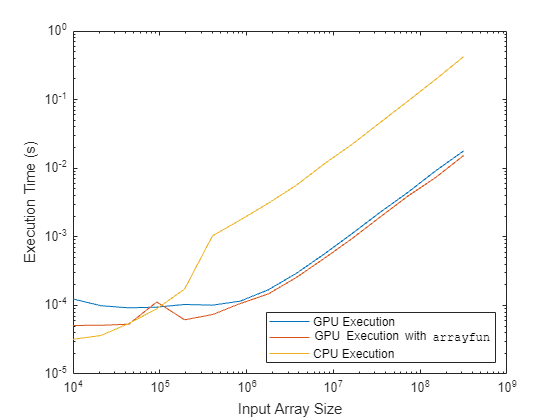# Improve Performance of Element-Wise MATLAB Functions on the GPU Using `arrayfun`

This example shows how to improve the performance of your code by running MATLAB® functions on the GPU using `arrayfun`.

When a MATLAB function contains many element-wise operations, using `arrayfun` can provide better performance than executing the MATLAB function directly on the GPU with `gpuArray` input data. For a function to be compatible with `arrayfun` it must be capable of operating on a single element of the input array to calculate a single element of the output array using scalar operations and arithmetic.

In this example, you compare the execution times of a function executing on the CPU, on the GPU without using `arrayfun`, and on the GPU using `arrayfun`.

### Define Function for Testing

The Lorentz factor determines how, according to the principles of special relativity, the physical properties of an object change while that object is moving. For an object moving at a velocity $\mathit{v}$ relative to an observer, the Lorentz factor $\gamma$ is defined as

$\gamma =\frac{1}{\sqrt{1-\frac{{\mathit{v}}^{2}}{{\mathit{c}}^{2}}}}=\frac{1}{\sqrt{1-{\beta }^{2}}}$.

$\beta$ is the ratio of $\mathit{v}$ to $\mathit{c}$, where $\mathit{c}$ is the speed of light in a vacuum. The `lorentz` function, defined at the end of the example, calculates the Lorentz factor as follows.

```Y = 1./sqrt(1-B.*B); ```

### Prepare Function for GPU Execution

Most MATLAB functions execute on the CPU by default. To execute `lorentz` on the GPU, provide a `gpuArray` object as input. `gpuArray` objects represent an array stored in GPU memory. Because many functions support `gpuArray` inputs, you can often run your code on a GPU with minimal changes to the code. For more information see, Run MATLAB Functions on a GPU.

Because `lorentz` contains individual element-wise operations, performing each operation one at a time on the GPU does not yield significant performance improvements. You can improve the performance by executing all of the operations in the `lorentz` function at once using `arrayfun`.

To run the `lorentz` function on the GPU using `arrayfun`, define a handle to the function.

`lorentzFcn = @lorentz;`

### Set Comparison Parameters

In this example, you will compare execution times of the `lorentz` function operating on arrays containing${10}^{4}$ to ${10}^{8.5}$ elements. Set the number of comparisons to run, and the upper and lower limits on the size of the arrays to pass to the function.

```numComp = 15; lowerLimit = 4; upperLimit = 8.5;```

Specify the size of the arrays to pass to the function as a logarithmically spaced array. This example can take several minutes to run. To reduce the execution time, reduce the upper limit on the array size.

`arraySize = ceil(logspace(lowerLimit,upperLimit,numComp));`

### Time Execution on the CPU and the GPU

To time the different modes of execution:

1. Generate random, single-precision input data of increasing sizes.

2. Time how long `lorentz` takes to execute on the CPU using `timeit`.

3. Send the input data to the GPU using `gpuArray`.

4. Time the duration of executing `lorentz` on the GPU using `gputimeit`.

5. Time the duration of executing `lorentz` on the GPU using `arrayfun` using `gputimeit`.

```for i = 1:numComp fprintf('Timing function for input array of size %d \n', arraySize(i)); % Create random input data data = rand(arraySize(i),1,"single"); % CPU execution tcpu(i) = timeit(@() lorentzFcn(data)); % Send data to the GPU gdata = gpuArray(data); % GPU execution using only gpuArray objects tgpuObject(i) = gputimeit(@() lorentzFcn(gdata)); % GPU execution using gpuArray objects with arrayfun tgpuArrayfun(i) = gputimeit(@() arrayfun(lorentzFcn,gdata)); end```
```Timing function for input array of size 10000 Timing function for input array of size 20962 Timing function for input array of size 43940 Timing function for input array of size 92106 Timing function for input array of size 193070 Timing function for input array of size 404709 Timing function for input array of size 848343 Timing function for input array of size 1778280 Timing function for input array of size 3727594 Timing function for input array of size 7813708 Timing function for input array of size 16378938 Timing function for input array of size 34333201 Timing function for input array of size 71968568 Timing function for input array of size 150859071 Timing function for input array of size 316227767 ```

### Compare Results

To compare the results, plot the execution times against the number of data elements.

```loglog(arraySize,[tgpuObject; tgpuArrayfun; tcpu]) xlabel("Input Array Size") ylabel("Execution Time (s)") legend(["GPU Execution" "GPU Execution with \fontname{courier}arrayfun" "CPU Execution"], ... location="southeast")```For smaller arrays, the CPU executes the function faster than the GPU. As the size of the input array increases, the performance of the GPU improves relative to the performance of the CPU. Above a threshold array size, the GPU executes the function faster than the CPU. The threshold at which GPU performance exceeds CPU performance depends on the hardware that you use and the function that you execute.

Calculate the ratio of CPU execution time to GPU execution time only and to GPU execution time using `arrayfun` respectively.

```gpuObjectSpeedup = tcpu./tgpuObject; gpuArrayfunSpeedup = tcpu./tgpuArrayfun;```

Plot the ratios against the input array size.

```semilogx(arraySize,[gpuObjectSpeedup;gpuArrayfunSpeedup]) xlabel("Input Array Size") ylabel("Ratio of CPU to GPU Execution Times") legend(["GPU Execution" "GPU Execution with \fontname{courier}arrayfun"],location="southeast")```Executing `lorentz` using `arrayfun` is consistently faster than execution on the GPU only. When you apply the techniques described in this example to your own code, the performance improvement will strongly depend on your hardware and on the code you run.

### Supporting Functions

The `lorentz` function takes $\beta$ and calculates the Lorentz factor according to this equation

$\gamma =\frac{1}{\sqrt{1-\frac{{\mathit{v}}^{2}}{{\mathit{c}}^{2}}}}=\frac{1}{\sqrt{1-{\beta }^{2}}}$.

```function Y = lorentz(B) Y = 1./sqrt(1-B.*B); end```